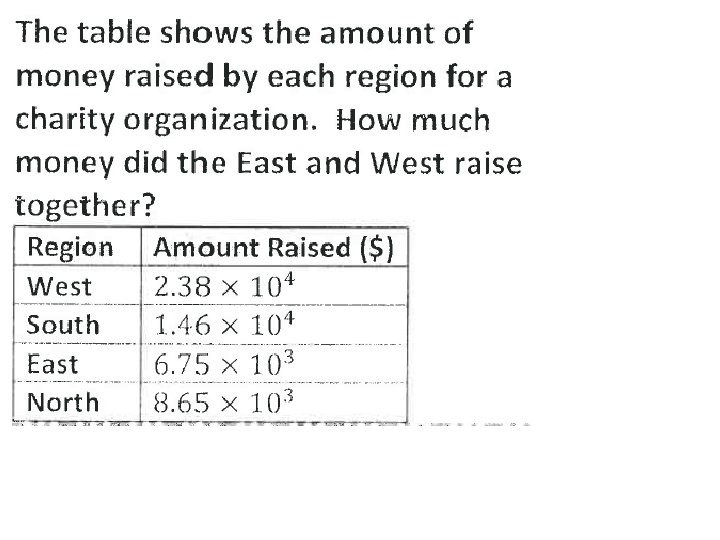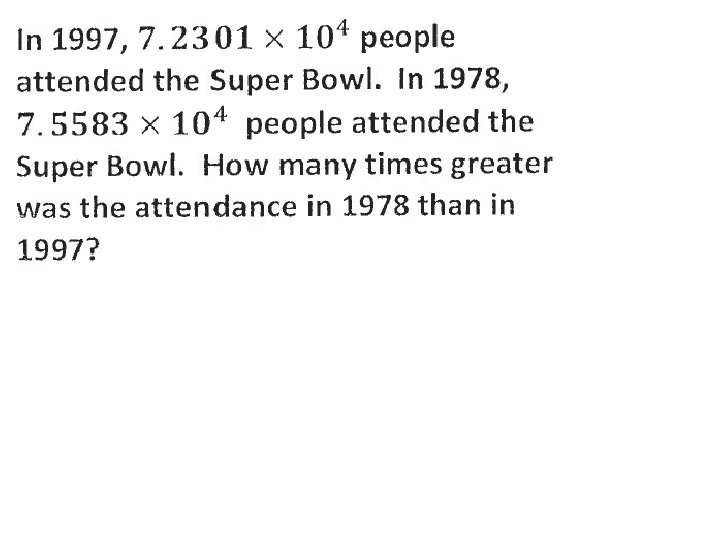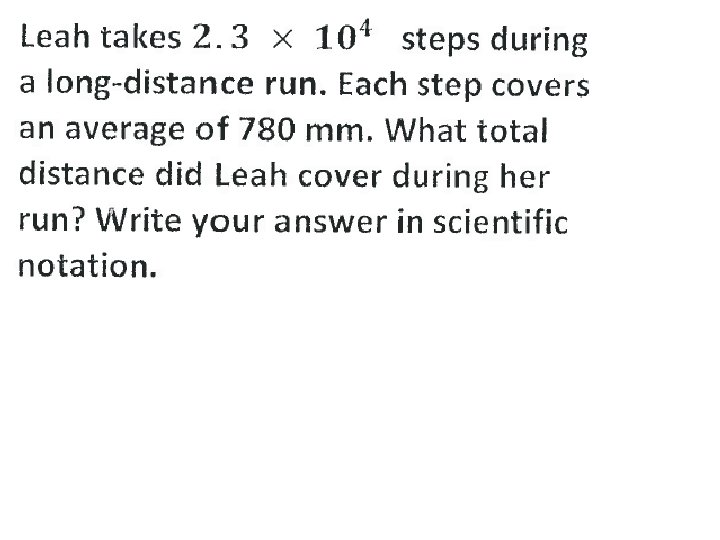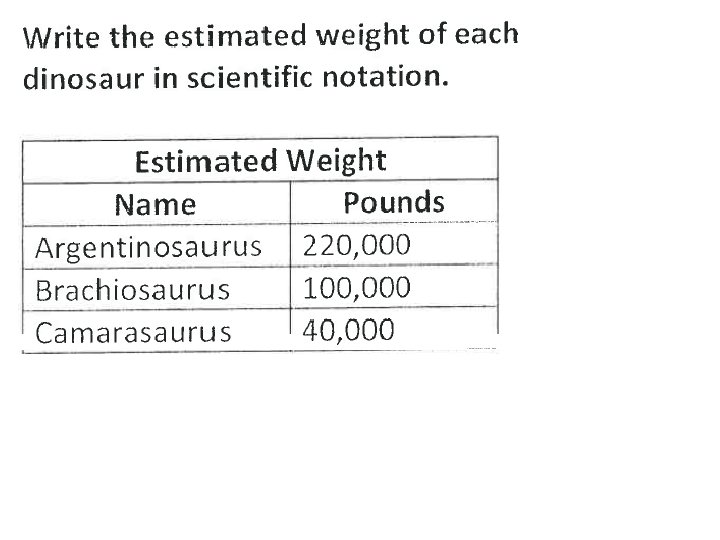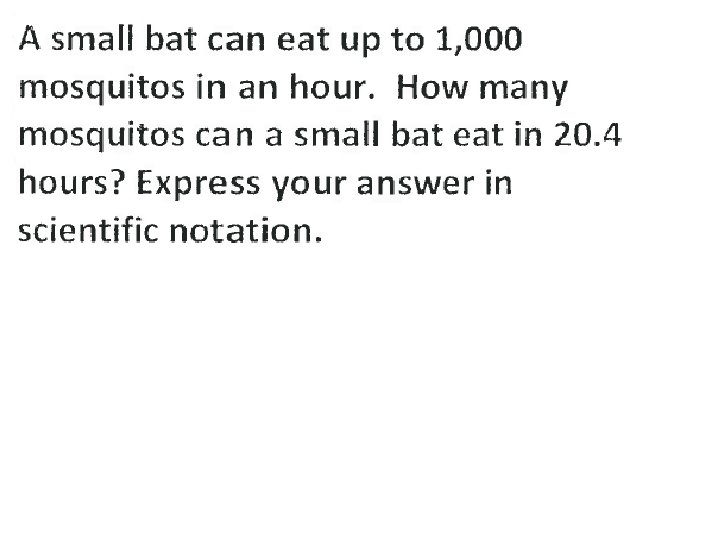# Scientific Notation Problem Solving 8 44 Scientific notation

• Slides: 20Scientific Notation Problem Solving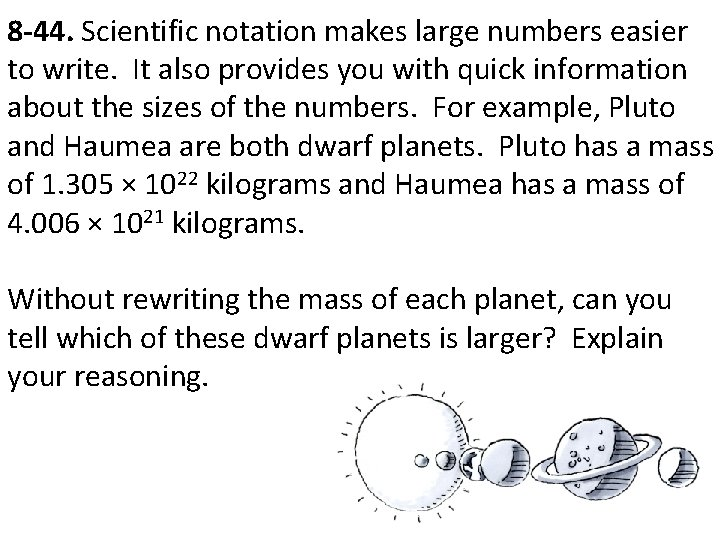8 -44. Scientific notation makes large numbers easier to write. It also provides you with quick information about the sizes of the numbers. For example, Pluto and Haumea are both dwarf planets. Pluto has a mass of 1. 305 × 1022 kilograms and Haumea has a mass of 4. 006 × 1021 kilograms. Without rewriting the mass of each planet, can you tell which of these dwarf planets is larger? Explain your reasoning.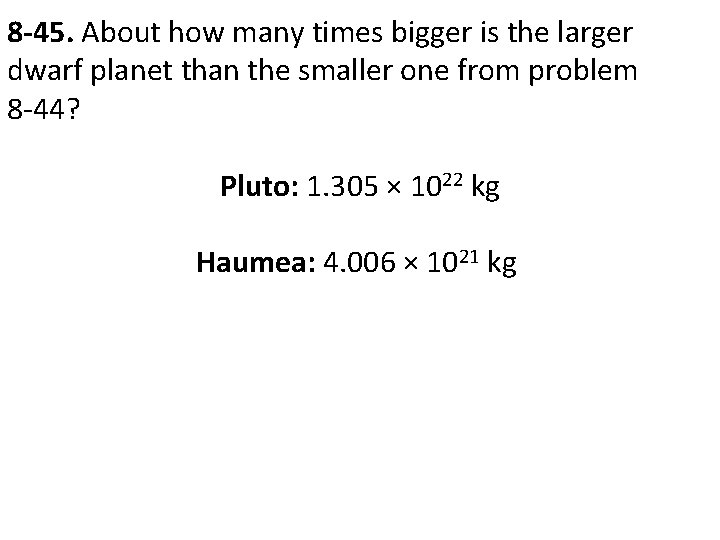8 -45. About how many times bigger is the larger dwarf planet than the smaller one from problem 8 -44? Pluto: 1. 305 × 1022 kg Haumea: 4. 006 × 1021 kg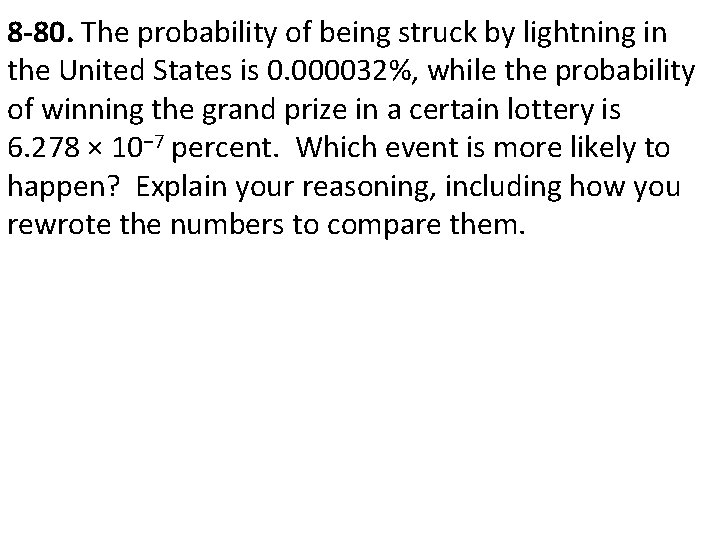8 -80. The probability of being struck by lightning in the United States is 0. 000032%, while the probability of winning the grand prize in a certain lottery is 6. 278 × 10− 7 percent. Which event is more likely to happen? Explain your reasoning, including how you rewrote the numbers to compare them.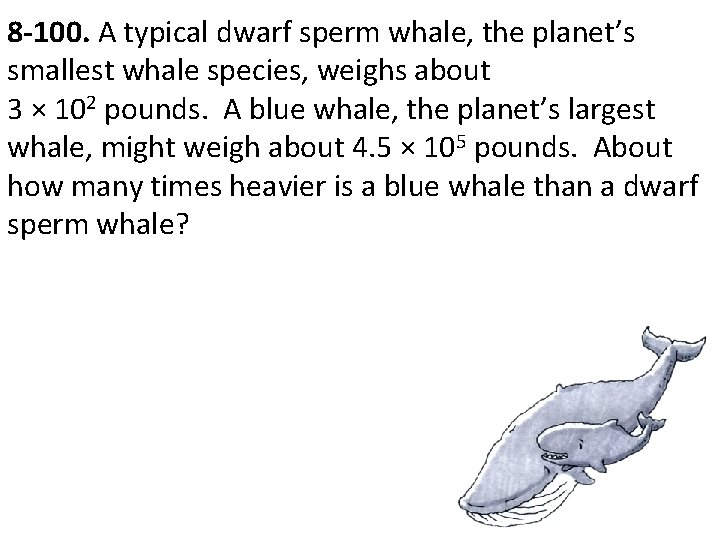8 -100. A typical dwarf sperm whale, the planet’s smallest whale species, weighs about 3 × 102 pounds. A blue whale, the planet’s largest whale, might weigh about 4. 5 × 105 pounds. About how many times heavier is a blue whale than a dwarf sperm whale?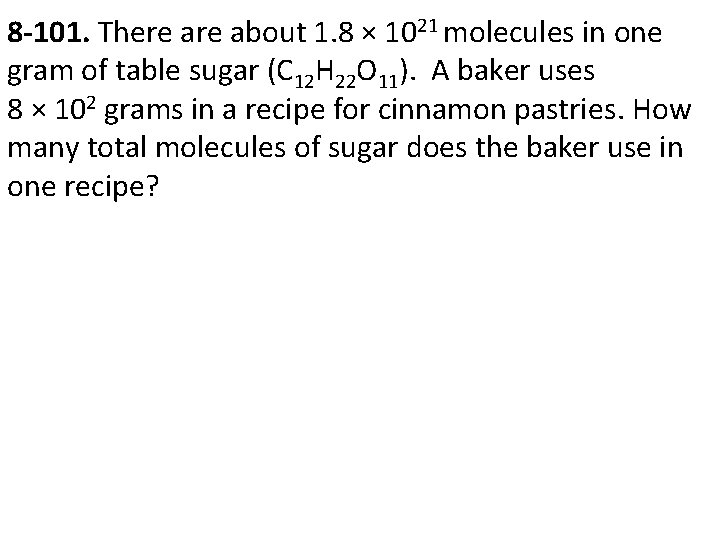8 -101. There about 1. 8 × 1021 molecules in one gram of table sugar (C 12 H 22 O 11). A baker uses 8 × 102 grams in a recipe for cinnamon pastries. How many total molecules of sugar does the baker use in one recipe?8 -103. The average distance from the sun to the earth is 1. 488 × 1011 meters. If the length of the average paperclip is 3 × 10− 2 meters, how many paperclips would need to be connected together to reach the sun?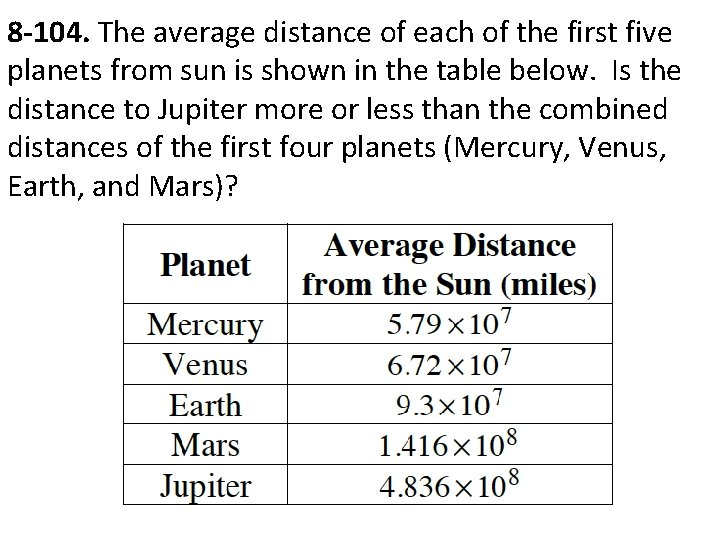8 -104. The average distance of each of the first five planets from sun is shown in the table below. Is the distance to Jupiter more or less than the combined distances of the first four planets (Mercury, Venus, Earth, and Mars)?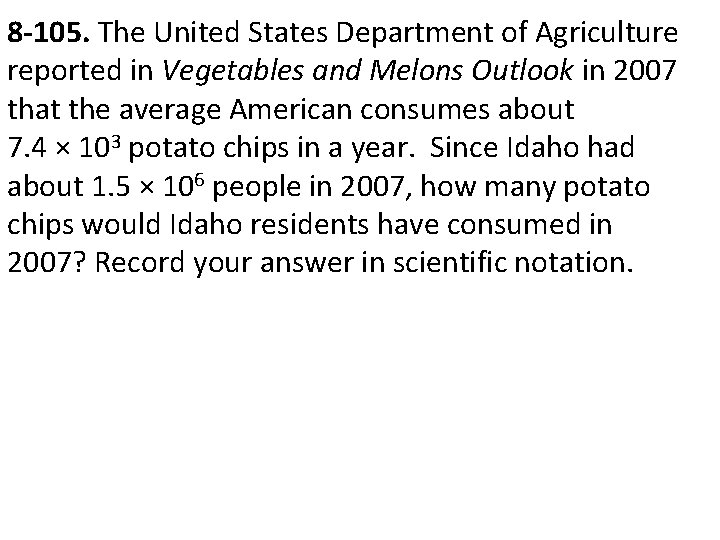8 -105. The United States Department of Agriculture reported in Vegetables and Melons Outlook in 2007 that the average American consumes about 7. 4 × 103 potato chips in a year. Since Idaho had about 1. 5 × 106 people in 2007, how many potato chips would Idaho residents have consumed in 2007? Record your answer in scientific notation.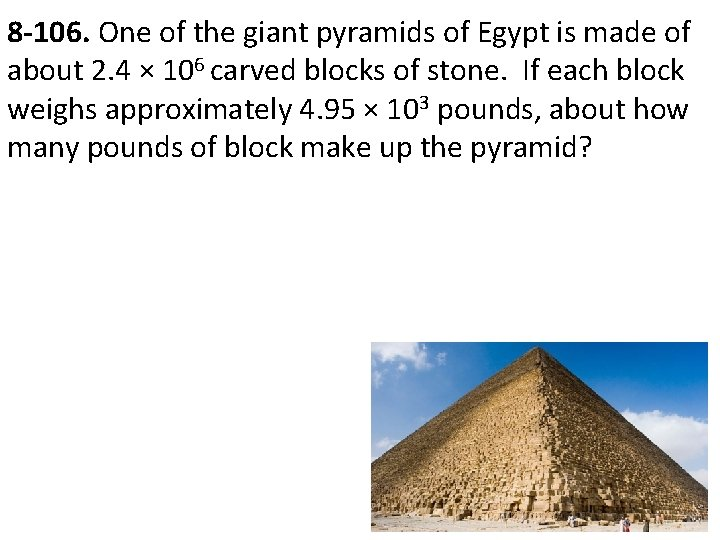8 -106. One of the giant pyramids of Egypt is made of about 2. 4 × 106 carved blocks of stone. If each block weighs approximately 4. 95 × 103 pounds, about how many pounds of block make up the pyramid?